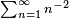# Basel problem

The Basel problem is a question about infinite series, first formulated by Pietro Mengoli in 1650 Many of the most talented and influential mathematicians of that time attempted and failed to solve the problem. Its solution was one of the early triumphs of Leonard Euler, and immediately made him renown in European mathematical circles.

Mengoli knew that the series of positive numbers$\sum_{n=1}^{\infty} n^{-2}$ is convergent. In the Basel problem, he asked for its sum. Euler showed that$\sum_{k=1}^{\infty} \frac{1}{n^2} = \frac{\pi^2}{6}.$

He actually showed considerable more than this, evaluating the sums of several related series which, from a modern viewpoint, are values of the Riemann zeta function at positive even integers. His argument was highly non-rigorous, assuming that results known to be true for finite sums and products also extend to infinite series and products. Even so, he had already calculated its value to 20 decimal places, and up to this precision, the value matched his claimed π2 / 6. Thus, mathematicians of the time who disbelieved the validity of his argument could not argue that the value he had found was incorrect.